#### 3.1.1Variational Form

As a starting point, consider the boundary value problems (BVP) described by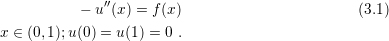Additionally, define a linear space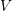as:

V = {v: continous functions in [0,1] with v’ piecewise continous and bounded in [0,1], and v(0)=v(1)=0} Along this session, it will be shown how the spacecan be used to reformulate the problem (3.1). From this new version, a numerical method will be developed based on the particular definition of, in order to obtain an approximate solution of (3.1). To begin, take an element of the space, multiply by (3.1) and integrate over the entire domain as in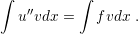(3.2)
The functionis known as a test function. Initially, it is not clear how (3.2) can help to solve (3.1), but it provides a different view of the problem (3.1). Indeed, it is possible to simplify (3.2) by integrating the left hand side by parts, according to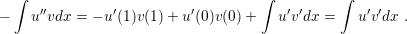(3.3)
Subsequently, substituting the results from (3.3) in (3.1) the relation holds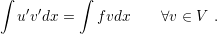(3.4)
(3.4) is known as the variational formulation of the problem (3.1). Variational formulations can be handled by several numerical methods, and it is usually easier to prove the existence of the solution of a variational problem in comparison to a PDE. Seemingly, the solution of (3.1) solves (3.4), but to be useful, the variational formulation should work in the opposite direction, where the solution of (3.4) solves (3.1). This is true for the form described by (3.4), but usually the methods applied to solve variational problems impose some restriction in the solution, which should also satisfy (3.1).